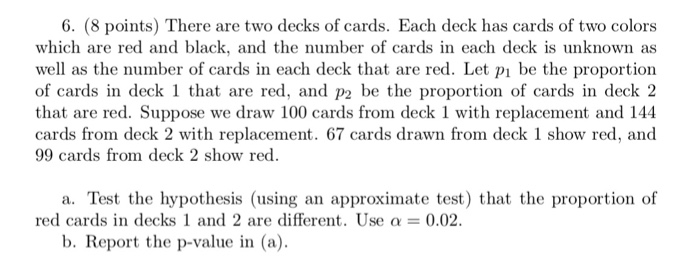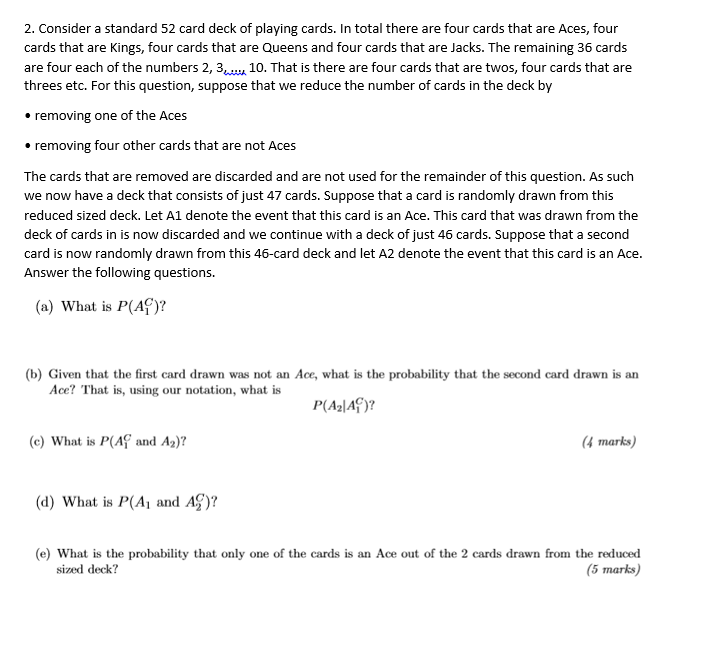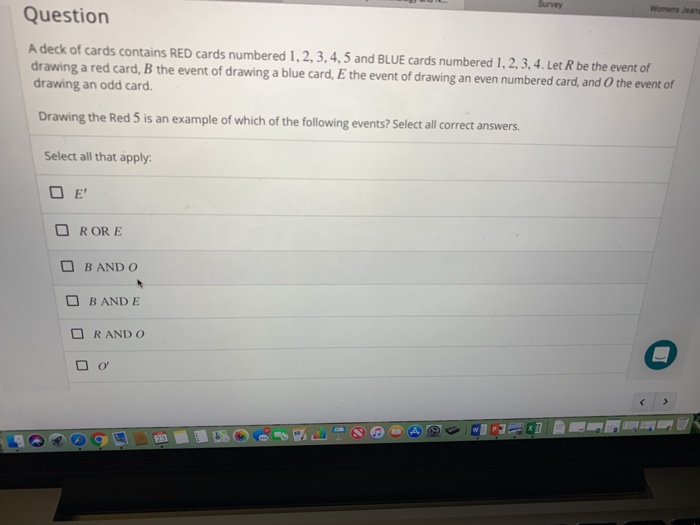# Construct a probability distribution for the following: (a) Construct a probability distribution for drawing a card from a deck of 40 cards consisting of 10 cards numbered 1, 10 cards numbered 2, 15 cards numbered 3, and 5 cards numbered 4 related homework questions

• #### Construct a probability distribution for the following: (a) Construct a probability distribution for drawing a card from a deck of 40 cards consisting of 10 cards numbered 1, 10 cards numbered 2, 15 cards numbered 3, and 5 cards numbered 4

Construct a probability distribution for the following: (a) Construct a probability distribution for drawing a card from a deck of 40 cards consisting of 10 cards numbered 1, 10 cards numbered 2, 15 cards numbered 3, and 5 cards numbered 4. (b) Using the sample space for tossing two dice, construct a probability distribution for the sum 2 through 12.

• #### It’s review question, I need this as soon as possible. Thank you 3) For thè diferential equation: (a) The point zo =-1 is an ordinary point. Compute the recursion formula for the coefficients of...It’s review question, I need this as soon as possible. Thank you 3) For thè diferential equation: (a) The point zo =-1 is an ordinary point. Compute the recursion formula for the coefficients of the power series solution centered at zo- -1 and use it to compute the first three nonzero terms of the power series when -1)-s and v(-1)-0....

• #### Dont copié formé thé book oh ya dont copié formé thé book cause you Oiil inde up being triste soi remembré not toi copié frome thé book oh ya

Dont copié formé thé book oh ya dont copié formé thé book cause you Oiil inde up being triste soi remembré not toi copié frome thé book oh ya!translation in english please!

• #### Event A represents the act of drawing a rank of eight from a standard deck of 52 cards, if B represents the event of drawing any diamond after the first card has been replaced into the deck, what is the probability of A and B

Event A represents the act of drawing a rank of eight from a standard deck of 52 cards, if B represents the event of drawing any diamond after the first card has been replaced into the deck, what is the probability of A and B.

• #### 6. (8 points) There are two decks of cards. Each deck has cards of two colors which are red and black, and the number of cards in each deck is unknown as well as the number of cards in each deck...6. (8 points) There are two decks of cards. Each deck has cards of two colors which are red and black, and the number of cards in each deck is unknown as well as the number of cards in each deck that are red. Let pi be the proportion of cards in deck 1 that are red, and p2 be...

• #### DSuppose \$39oo is deposited in a savings account that increases exponentially.Detamine thě APv if the acount...DSuppose \$39oo is deposited in a savings account that increases exponentially.Detamine thě APv if the acount increases to \$t020 in 4 years. Ass ume tne interest Vale remains Constant and no additional deposits or Withdrawals are made. (a.) Let pbe the APY. Note tnat if tme inital balaqe is yo, ne year later tne balane is %more. P- 3 (Tpe...

• #### 2. Consider a standard 52 card deck of playing cards. In total there are four cards that are Aces, four cards that are Kings, four cards that are Queens and four cards that are Jacks. The remaining 3...2. Consider a standard 52 card deck of playing cards. In total there are four cards that are Aces, four cards that are Kings, four cards that are Queens and four cards that are Jacks. The remaining 36 cards are four each of the numbers 2, 310. That is there are four cards that are twos, four cards that are...

• #### Consider the experiment of selecting a card from an ordinary deck of 52 playing cards and determine the probability of a red card or a card showing a 5 is drawn

Consider the experiment of selecting a card from an ordinary deck of 52 playing cards and determine the probability of a red card or a card showing a 5 is drawn.I figured the red cards would be 26/52 and the 5 would be 4/52. Would I add these two together for the answer of 28/52 or do I keep these...

• #### Question A deck of cards contains RED cards numbered 1,2, 3, 4,5 and BLUE cards numbered...Question A deck of cards contains RED cards numbered 1,2, 3, 4,5 and BLUE cards numbered 1, 2,3,4. Let R be the event of drawing a red card, B the event of drawing a blue card, E the event of drawing an even numbered card, and O the event of drawing an odd card. Drawing the Red 5 is an...

• #### Question A deck of cards contains RED cards numbered 1,2,3,4 and BLUE cards numbered 1,2,3, as...

Question A deck of cards contains RED cards numbered 1,2,3,4 and BLUE cards numbered 1,2,3, as shown below. Let R be the event of drawing a red card, B be the event of drawing a blue card, E be the event of drawing an even numbered card, and O be the event of drawing an odd numbered card. Drawing the...

• #### If one card is drawn from a standard deck of 52 playing cards, what the probability of drawing is a red card?

Need Online Homework Help?